# 1- Optimization algorithms

3. 引入标记：使用上标{i}表示拆分出的第i个批次。比如 $$X^{\{i\}}$$ 表示第i个批次组成的数据集。如果一个批次的大小是1000，则每个批次的数据集$$X^{\{i\}}$$ 的shape都是$$(n_x, 1000)$$ ， Y的shape是(1, 1000)（不考虑最后一个批次零头的情况）。
4. mini-batch算法很简单：
• 先确定分多个batch，假如总的数据集大小是500万，分成5000个批去做，那么每个批处理1000个数据集。循环5000个批次：
5. epoch：当所有批次都循环完成了，即全部m个数据集都参与过了计算，这叫一个epoch。整个算法在epoch层还会做循环，迭代很多次epoch。
7. 相比而言，mini-batch gradient descent的迭代过程会比较震荡。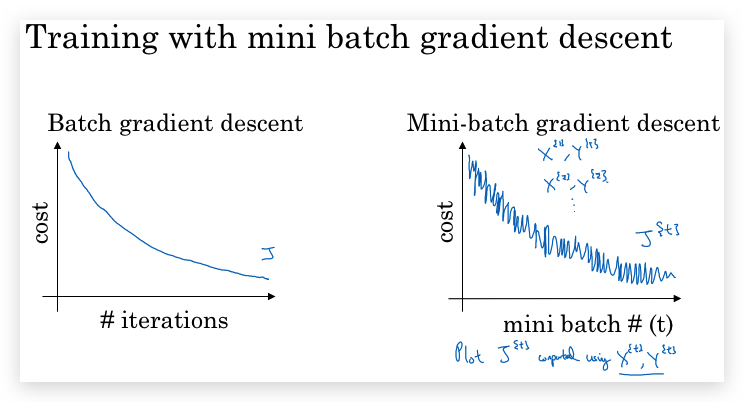8. 不同mini-batch size（批次大小，即一个批次包含的样本数）的区别：
• 如果mini-batch size = 1，则每次只用一个样本做梯度下降，则变成 stochastic gradient descent （随机梯度下降）。噪声太大，且失去了vectorization加速。
• mini-batch和stochastic都存在噪声问题，且在local optima附近会徘徊。但设置合适大小的mini-batch size，噪声和徘徊问题可接受的范围内。
9. 如何选择mini-batch size（这是一个hyperparameter）：
• 大数量，mini-batch size倾向于选择2^n个，比如64, 128, 256等
• mini-batch 与CPU/GPU memory的内存容量。

## 1-2 Exponentially weighted averages

Exponentially weighted averages（指数加权平均），在统计学也称作Exponentially weighted moving averages（指数加权滑动平均）

### Exponentially weighted averages概念举例：

1. 曲线越平滑
2. 曲线会比实际值向右偏移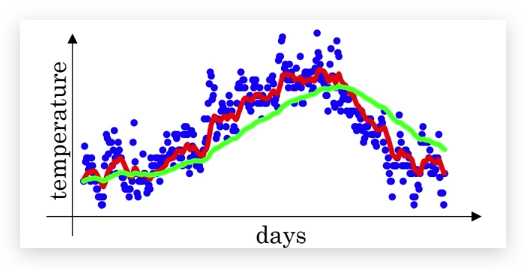### 如何理解Exponentially weighted average

$$v_t$$近似的等于最近$$\frac{1}{1-\beta}$$天的温度加权平均值： $$v_t \approx avg(\frac{1}{1-\beta} days' temperature)$$ 比如β=0.9，则近似相当于最近10天的温度加权均值。

### 机器学习实践中的操作

1. 并不是无限制的计算所有历史值的加权平均，而是近似的做前$$\frac{1}{(1-\beta)}$$天的加权平均值。
2. 计算前$$\frac{1}{(1-\beta)}$$天的平均值，使用循环不断override的的方法，减少内存占用，只需一行代码： $$v := \beta v + (1 - \beta)\theta_t$$

## 1-3 Gradient descent with momentum

1. 问题背景：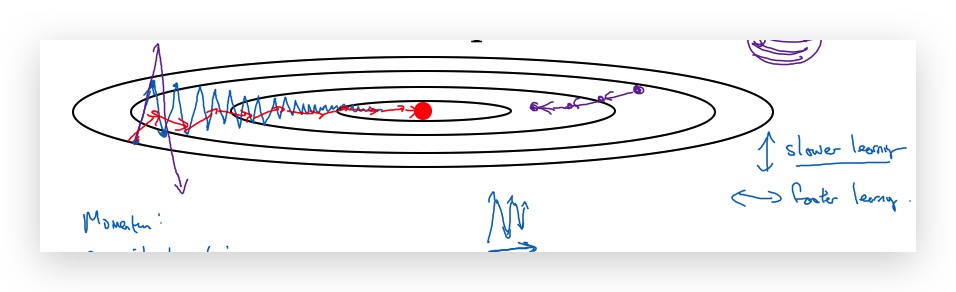• 某个方向（属性范围较小的，如上图蓝色曲线的垂直方向）出现震荡，会让梯度下降速度会变慢（Just slowly oscillate toward the minimum. And this up and down oscillations slows down gradient descent and prevents you from using a much larger learning rate.）
• 然而，又不能通过加大learning rate解决，因为这样会在出现overshooting（如上图左边的紫色曲线）。
2. 应用exponentially weighted average
• 与上面伦敦气温类似，这里将每轮迭代的梯度做exponentially weighted average处理
• 每次梯度迭代（下面的式子省略标注参数所在的layer，另外$$v_{dW}$$和$$v_{dW}$$初始化为0）: $$v_{dW} := \beta v_{dW} + (1 - \beta) dW$$ $$v_{db} := \beta v_{db} + (1 - \beta) db$$ $$w=w - \alpha v_{dW}$$ $$b=b - \alpha v_{db}$$ 新的算法使用$$v_{dW}$$和$$v_{dW}$$代替了原始的梯度。这样，可以让gradient更平滑
• 对于上图垂直方向，原来是会上下震荡，但引入了exponentially weighted average，相当于对前面的震荡进行了平均，结果就是上下震荡互相抵消了。而水平方向都是向右没有震荡，因此平均后还是向右。最终导致呈现上图红色的下降路线。
3. Intuition for momentum 可以将上面的图想象成一个碗，梯度下降就像一个小球往碗底滚，而β的作用就相当于摩擦力
4. 引入了超参：β，实践中通常取0.9。
5. 通常并不像上面计算温度的时候需要做bias correction，因为对梯度下降来说迭代次数很多，初期的不准确影响并不大，如果β为0.9，大概10次就看不到bias了。
6. 另外，对于抑制mini-batch的震荡也有很好的效果： Because mini-batch gradient descent makes a parameter update after seeing just a subset of examples, the direction of the update has some variance, and so the path taken by mini-batch gradient descent will “oscillate” toward convergence. Using momentum can reduce these oscillations. 换个角度理解：在mini-batch中，引入之前迭代gradient做平均，相当于变相考虑了全部的数据集的特征。

## 1-4 RMSprop

RMSprop (Root Mean Square Propagation，均方根传递)，与momentum一样，也是降低梯度的抖动。以上面的图为例，降低处置方向的下降速率，并提升水平方向的下降速率。

1. 算法： $$s_{dw} = \beta s_{dw} + (1 - \beta)(dw)^2$$ $$s_{db} = \beta s_{db} + (1 - \beta)(db)^2$$ $$w := w - \alpha \frac{dw}{\sqrt{s_{dw} + \varepsilon}}$$ $$b := b - \alpha \frac{db}{\sqrt{s_{db} + \varepsilon}}$$

1. Intuition 垂直方向，比较陡，梯度比较大，但我们又希望它下降的慢。因此对梯度除以一个较大的值，所以用梯度的平方的平均来表示。让不同的参数拥有不同的learning rate。

1. 引入的变量有：
• $$v$$ : 计算同momentum算法，将梯度进行指数加权平均
• $$s$$ : 计算同RMSprop，将梯度的平方进行指数加权平均
• $$\beta_1$$ : 计算$$v$$的加权参数
• $$\beta_2$$ : 计算$$s$$的加权参数
2. 在迭代前，初始化参数v和s $$v_{dW} = 0, s_{dW} = 0, v_{db} = 0, s_{db} = 0$$

3. 对第t次梯度下降的迭代 a. 首先计算dw和db的v和s $$v_{dW} = \beta_1 v_{dW} + (1 - \beta_1) dW$$ $$s_{dW} = \beta_2 s_{dW} + (1 - \beta_2) (dW)^2$$ $$v_{db} = \beta_1 v_{db} + (1 - \beta_1) db$$ $$s_{db} = \beta_2 s_{db} + (1 - \beta_2) (db)^2$$

b. 然后做修正

$$v^{corrected}\_{dW} = \frac{v_{dW}}{1 - (\beta_1)^t}$$ $$s^{corrected}\_{dW} = \frac{s_{dW}}{1 - (\beta_2)^t}$$ $$v^{corrected}\_{db} = \frac{v_{db}}{1 - (\beta_1)^t}$$ $$s^{corrected}\_{db} = \frac{s_{db}}{1 - (\beta_2)^t}$$

c. 最后更新参数W和b

$W = W - \alpha \frac{v^{corrected}\_{dW}}{\sqrt{s^{corrected}\_{dW}} + \varepsilon}$ $b = b - \alpha \frac{v^{corrected}\_{db}}{\sqrt{s^{corrected}\_{db}} + \varepsilon}$

## 1-6 Learning rate decay

• 倒数： $$\alpha := \frac{1}{1 + decay\\_rate * epoch\\_num} \alpha$$

• 指数函数： $$\alpha := 0.95^{epoch\\_num} * \alpha$$

• 根号倒数 $$\alpha := \frac{k}{\sqrt{epoch\_num}} * \alpha$$

## 1-10 The problem of local optima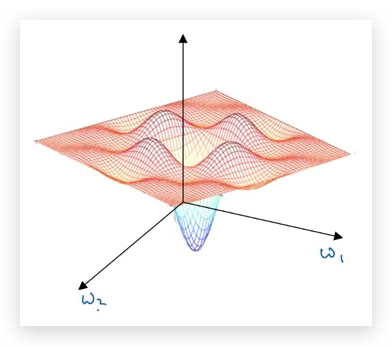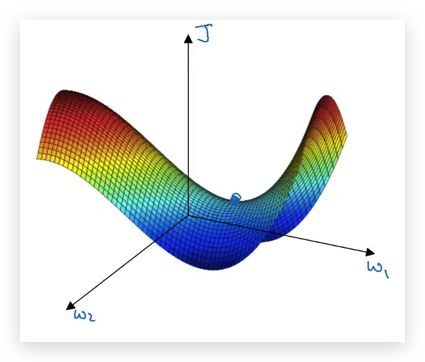-------------------------